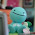## Thursday, January 13, 2011

### Final Percent Post

What is a Percent?
Percent is a number out of 100.
For example 50% is 50 out of 100.

Percent can be turned into a decimal and to a fraction.
For example 50% in decimal will be 0.5 and in fraction it's going to be 1/2.

If your turning a percent to a decimal, divide the percent by 100 and you'll get your answer

If your turning a percent to a fraction first find the decimal of the percent, second count how many digits you have after the decimal point then put zero's every digit you have after the decimal.
E.G 45%=0.45=45/100

Percents can be represent also by shading square from a hundred grid. To represent 100% in to a hundred grid color all of the squares. To represent a percent that is less than 1% shade part of one square then draw another square and divide that square whatever is the denominator given to you.

Percent have a strategies like halving, doubling, and dividing by ten if you are to ask use mental math.

Here is my video for the percent questions

#### 1 comment:

1.Good job Alec G. I like how you used some colour but not a lot maybe you can change that. I also like how you stuck with one font, maybe next time you can bold some things out so that it can be easier.